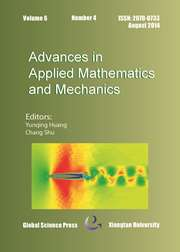Home
Hostname: page-component-544b6db54f-8tjh8 Total loading time: 0.168 Render date: 2021-10-23T23:55:33.141Z Has data issue: true Feature Flags: { "shouldUseShareProductTool": true, "shouldUseHypothesis": true, "isUnsiloEnabled": true, "metricsAbstractViews": false, "figures": true, "newCiteModal": false, "newCitedByModal": true, "newEcommerce": true, "newUsageEvents": true }Advances in Applied Mathematics and Mechanics

# Tailored Finite Point Method for Numerical Solutions of Singular Perturbed Eigenvalue Problems

Published online by Cambridge University Press:  03 June 2015

*
Corresponding author. Email: yintzer shih@nchu.edu.tw

## Abstract

We propose two variants of tailored finite point (TFP) methods for discretizing two dimensional singular perturbed eigenvalue (SPE) problems. A continuation method and an iterative method are exploited for solving discretized systems of equations to obtain the eigen-pairs of the SPE. We study the analytical solutions of two special cases of the SPE, and provide an asymptotic analysis for the solutions. The theoretical results are verified in the numerical experiments. The numerical results demonstrate that the proposed schemes effectively resolve the delta function like of the eigenfunctions on relatively coarse grid.

Type
Research Article
Information

## Access options

Get access to the full version of this content by using one of the access options below. (Log in options will check for institutional or personal access. Content may require purchase if you do not have access.)

## References

Allgower, E. L. AND Georg, K., An Introduction to Numerical Continuation Methods, SIAM Publications, Philadelphia, 2003.CrossRefGoogle Scholar
Avila, A. and Jeanjean, L., A result on singularly perturbed elliptic problems, Commun. Pure App. Anal., 4.2 (2005), pp. 343358.Google Scholar
Babuska, I. AND Osborn, J. E., Eigenvalue Problems, Handbook of Numerical Analysis, Finite Element Methods (Part 1), Vol. 2, pp. 641787, Ed. by Ciarlet, P. G. and Lious, J. L., Amsterdam, 1991.CrossRefGoogle Scholar
Golub, G. H. AND Van Loan, C. F., Matrix Computations, 3rd ed., Johns Hopkins University Press, 1996.Google Scholar
Han, H. AND Huang, Z., Tailored finite point method for a singular perturbation problem with variable coefficients in two dimensions, J. Sci. Comput., 41 (2009), pp. 200220.CrossRefGoogle Scholar
Han, H. AND Huang, Z., Tailored finite point method for steady-state reaction-diffusion equations, Commun. Math. Sci., 8 (2010), pp. 887899.CrossRefGoogle Scholar
Han, H. AND Huang, Z., Tailored finite point method based on exponential bases for convection- diffusion-reaction equation, Math. Comput., 82 (2013), pp. 213226.CrossRefGoogle Scholar
Han, H. and Huang, Z., A tailored finite point method for the Helmholtz equation withhighwave numbers in heterogeneous medium, J. Comput. Math., 26 (2008), pp. 728739.Google Scholar
Han, H., Huang, Z. and Kellogg, R. B., A tailored finite point method for a singular perturbation problem on an unbounded domain, J. Sci. Comput., 36 (2008), pp. 243261.CrossRefGoogle Scholar
Han, H., Miller, J. J. H. and Tang, M., A parameter-uniform tailored finite point method for singularly perturbed linear ODE systems, J. Comput. Math., 31 (2013), pp. 422438.CrossRefGoogle Scholar
Han, H., Tang, M. and Ying, W., Two uniform tailored finite point schemes for the two dimen-sional discrete ordinates transport equations with boundary and interface layers, Commun. Comput. Phys., 15 (2014), pp. 797826.CrossRefGoogle Scholar
Han, H., Zhou, Z. and Zheng, C., Numerical solutions of an eigenvalue problem in unbounded domains, Numer. Math. J. Chinese Univ. (English Ser.), 14 (2006), pp. 113.Google Scholar
Hsieh, P., Shih, Y. and Yang, S., A tailored finite point method for solving steady MHD duct flow problems with boundary layers, Commun. Comput. Phys., 10 (2011), pp. 161182.CrossRefGoogle Scholar
Huang, Z. AND Yang, X., Tailored finite point method for first order wave equation, J. Sci. Comput., 49 (2011), pp. 351366.CrossRefGoogle Scholar
Reed, M. AND Simon, B., Methods of Modern Mathematical Physics, IV: Analysis of Operators, Academic Press, Inc., 1978.Google Scholar
Schulten, K., Notes on Quantum Mechanics, Department of Physics and Beckman Institute, University of Illinois at UrbanaChampaign, 1999.Google Scholar
Shankar, R., Principles of Quantum Mechanics, 2nd Ed., New York, Kluwer Academic/Plenum Publishers, 1994.CrossRefGoogle Scholar
Shih, Y., Kellogg, R. B. and Chang, Y., Characteristic tailored finite point method for convection-dominated convection-diffusion-reaction problems, J. Sci. Comput., 47 (2011), pp. 198215.CrossRefGoogle Scholar
Shih, Y., Kellogg, R. B. and Tsai, P., A tailored finite point method for convection-diffusion- reaction problems, J. Sci. Comput., 43 (2010), pp. 239260.CrossRefGoogle Scholar
Szyld, D. B., Criteria for combining inverse and Rayleigh quotient iteration, SIAM J. Numer. Anal., 53 (1988), pp. 13691375.CrossRefGoogle Scholar
Parlett, B. N., The Symmetric Eigenvalue Problem, PrenticeHall, Englewood Cliffs, NJ, 1980.Google Scholar
4
Cited by

# Send article to Kindle

Note you can select to send to either the @free.kindle.com or @kindle.com variations. ‘@free.kindle.com’ emails are free but can only be sent to your device when it is connected to wi-fi. ‘@kindle.com’ emails can be delivered even when you are not connected to wi-fi, but note that service fees apply.

Find out more about the Kindle Personal Document Service.

Tailored Finite Point Method for Numerical Solutions of Singular Perturbed Eigenvalue Problems
Available formats
×

# Send article to Dropbox

To send this article to your Dropbox account, please select one or more formats and confirm that you agree to abide by our usage policies. If this is the first time you use this feature, you will be asked to authorise Cambridge Core to connect with your <service> account. Find out more about sending content to Dropbox.

Tailored Finite Point Method for Numerical Solutions of Singular Perturbed Eigenvalue Problems
Available formats
×

# Send article to Google Drive

To send this article to your Google Drive account, please select one or more formats and confirm that you agree to abide by our usage policies. If this is the first time you use this feature, you will be asked to authorise Cambridge Core to connect with your <service> account. Find out more about sending content to Google Drive.

Tailored Finite Point Method for Numerical Solutions of Singular Perturbed Eigenvalue Problems
Available formats
×
×